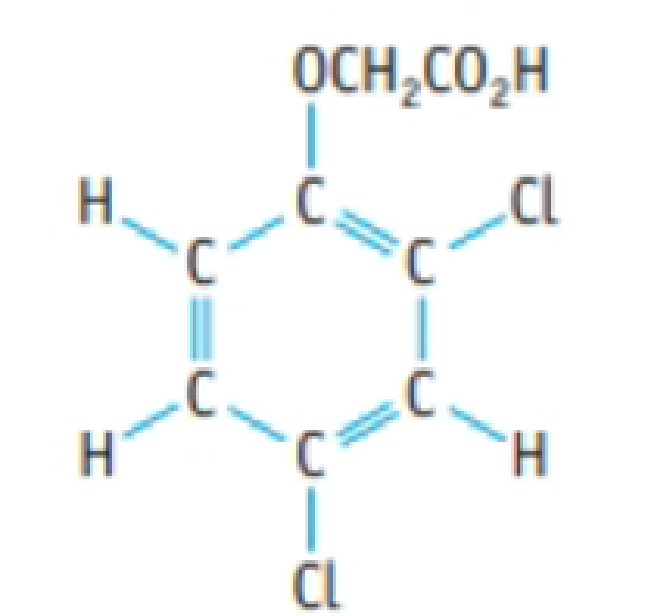Chapter 4, Problem 129IL

Chapter
Section
Textbook Problem

A herbicide contains 2,4-D (2,4-dichlorophenoxyacetic acid), C8H6Cl2O3. A 1.236-g sample of the herbicide was decomposed to liberate the chlorine as Cl− ion. This was precipitated as AgCl, with a mass of 0.1840 g. What is the mass percent of 2,4-D in the sample?2,4-D (2,4-dichlorophenoxyacetic acid)

Interpretation Introduction

Interpretation:

The mass percentage of 2,4-D(2,4-dichlorophenoxyaceticacid),C8H6Cl2O3 in the given sample of compound has to be determined.

Concept introduction:

• For chemical reaction balanced chemical reaction equation written in accordance with the Law of conservation of mass.
• Law of conservation of mass states that for a reaction total mass of the reactant and product must be equal.
• The molar mass of an element or compound is the mass in grams of 1 mole of that substance, and it is expressed in the unit of grams per mol (g/mol).
• Mass percent of elements of a compound is the ratio of mass of element to the mass of whole compound and multiplied with hundred.
• Numberofmole=GivenmassofthesubstanceMolarmass
Explanation

Here herbicide 2,4-D(2,4-dichlorophenoxyaceticacid) sample decomposed to liberate the chlorine as Cl ion. And it was precipitated as AgCl with a mass of 0.1840g

From the mass of  AgCl amount of 2,4-D(2,4-dichlorophenoxyaceticacid), and its mass can be calculated.

There is possibility to liberate 2molofCl from 1mol of C8H6Cl2O3.

Amount of AgCl produced in the reaction is,

0.1840g143.32g/mol=0.00128molAgCl

From this amount of AgCl the amount of C8H6Cl2O3 can be calculated as follows,

0

Still sussing out bartleby?

Check out a sample textbook solution.

See a sample solution

The Solution to Your Study Problems

Bartleby provides explanations to thousands of textbook problems written by our experts, many with advanced degrees!

Get Started

Each gene is a blueprint that directs the production of one or more of the body's organs. T F

Nutrition: Concepts and Controversies - Standalone book (MindTap Course List)

What gives amino acids their individual properties?

Biology: The Dynamic Science (MindTap Course List)

Define the following terms: a. chromosome b. chromatin

Human Heredity: Principles and Issues (MindTap Course List)

A body cavity fully lined with tissue derived from mesoderm is called a(n) _____.

Biology: The Unity and Diversity of Life (MindTap Course List)